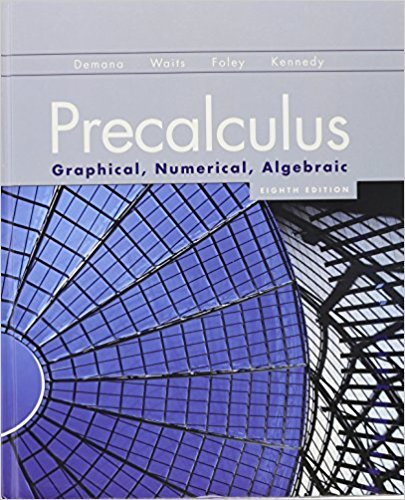×

×

# Solutions for Chapter 1.3: Functions and Graphs## Full solutions for Precalculus: Graphical, Numerical, Algebraic | 8th Edition

ISBN: 9780321656933Solutions for Chapter 1.3: Functions and Graphs

Solutions for Chapter 1.3
4 5 0 272 Reviews
27
5
##### ISBN: 9780321656933

This textbook survival guide was created for the textbook: Precalculus: Graphical, Numerical, Algebraic, edition: 8th Edition. Precalculus: Graphical, Numerical, Algebraic was written by and is associated to the ISBN: 9780321656933. This expansive textbook survival guide covers the following chapters and their solutions. Since 79 problems in chapter 1.3: Functions and Graphs have been answered, more than 94163 students have viewed full step-by-step solutions from this chapter. Chapter 1.3: Functions and Graphs includes 79 full step-by-step solutions.

Key Calculus Terms and definitions covered in this textbook
• Amplitude

See Sinusoid.

• Argument of a complex number

The argument of a + bi is the direction angle of the vector {a,b}.

• Descriptive statistics

The gathering and processing of numerical information

• Distance (on a number line)

The distance between real numbers a and b, or |a - b|

• Exponential form

An equation written with exponents instead of logarithms.

• Future value of an annuity

The net amount of money returned from an annuity.

• Gaussian elimination

A method of solving a system of n linear equations in n unknowns.

• Graph of parametric equations

The set of all points in the coordinate plane corresponding to the ordered pairs determined by the parametric equations.

• Heron’s formula

The area of ¢ABC with semiperimeter s is given by 2s1s - a21s - b21s - c2.

• Integers

The numbers . . ., -3, -2, -1, 0,1,2,...2

• Inverse composition rule

The composition of a one-toone function with its inverse results in the identity function.

• Inverse relation (of the relation R)

A relation that consists of all ordered pairs b, a for which a, b belongs to R.

• nth root of unity

A complex number v such that vn = 1

• One-to-one rule of logarithms

x = y if and only if logb x = logb y.

• Pie chart

See Circle graph.

• Power-reducing identity

A trigonometric identity that reduces the power to which the trigonometric functions are raised.

• Quotient polynomial

See Division algorithm for polynomials.

• Reciprocal of a real number

See Multiplicative inverse of a real number.

• Reference triangle

For an angle ? in standard position, a reference triangle is a triangle formed by the terminal side of angle ?, the x-axis, and a perpendicular dropped from a point on the terminal side to the x-axis. The angle in a reference triangle at the origin is the reference angle

• Square matrix

A matrix whose number of rows equals the number of columns.